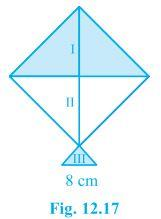# A kite in the shape of a square with a diagonal 32 cm and an isosceles triangle of base 8 cm and sides 6 cm each is to be made of three different shades as shown in Fig. How much paper of each shade has been used in it?As the area of the kite is in the square, its area will be

Area of kite = 1/2×(diagonal)2

= 1/2×32×32

= 512 cm2

Diagonal divides area into equal areas.

Area of kite = Area I + Area II

512 = 2 × Area I

The area I =Area II = 256 cm2

Area III: Area of triangle

Now, for each ar(△)can be calculated by Heron’s Formula, where

a = 6 cm

b = 6 cm

c = 8 cm

Semi Perimeter (s) = a+b+c/2

s = 6+6+8/2

s = 10 cm

ar(△) = √s(s-a)(s-b)(s-c)

= √10(10-6)(10-6)(10-8)

= √10×(4)×(4)×(2)

= 8√5 cm2